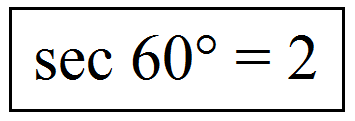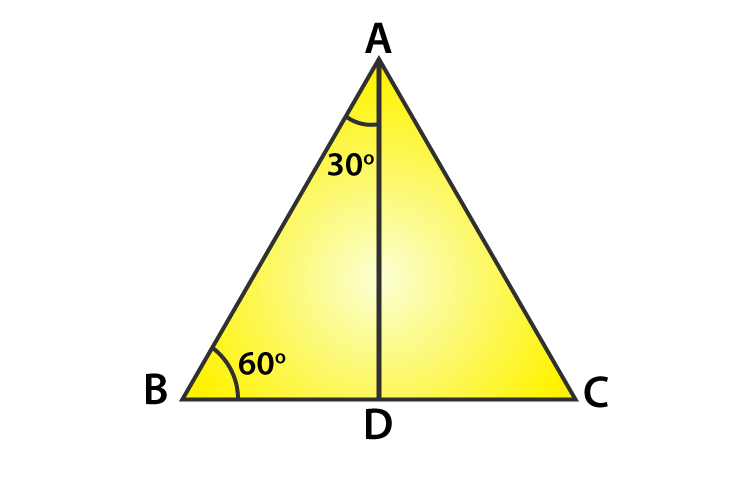Checkout JEE MAINS 2022 Question Paper Analysis : Checkout JEE MAINS 2022 Question Paper Analysis :

# Sec 60

The value of sec 60 degrees is 2.

The trigonometric functions are generally defined as an angle function that involves the study of triangles and relates the angles of a triangle and the dimension of the triangles. The trigonometric functions play an important role in our career, and trigonometric functions are used in various fields like engineering and architecture. In this article, let us discuss in detail the value for sec 60 degrees and how the values are derived geometrically.

The three most familiar trigonometric ratios are sine function, cosine function, and tangent function and the inverse function are cosecant function(cos), secant function(sec) and cotangent function(cot) respectively. For the angles less than a right angle where the trigonometric functions are defined as the ratio of two sides and an angle of a right triangle in which the values can be found the values obtained from the unit circle and sometimes the values can be obtained geometrically using triangle properties. Generally, the degrees are represented in the form of 0°, 30°, 45°, 60°, 90°, 180°, 270° and 360°.

## Sec 60 Degrees

The value of secant 60 degrees is equal to 2.To define the secant function of an acute angle, consider a right triangle ABC provided with the angle of interest and the sides of a triangle. The sides of the triangle are defined as follows:

• The side opposite to the angle of interest is stated as the opposite side.
• The longest side of a right triangle is called the hypotenuse side, which is the opposite side of the right angle.
• The side in which both the angle of interest and the right angle forms is called the adjacent side, and it is the remaining side of a triangle

The secant function is the reciprocal of the cosine function, and the sec function of an angle is defined as a ratio of the length of the hypotenuse side to the length of the adjacent side, and the formula is given by

Sec θ = 1 / cos θ

Therefore,

Sec θ = Hypotenuse Side / Adjacent Side

Since cosine function is defined as

Cos θ = Adjacent Side / Hypotenuse Side

## Derivation to Find Sec 60 Degree Value

Let us now calculate the value of sec 60°. Consider an equilateral triangle ABC. Since each angle in an equilateral triangle is 60°, therefore A = B = C=60°From the figure, draw the perpendicular line AD from A to the side BC

Now   ABD≅ ACD

So, BD=DC and also

It is observed that the triangle ABD is a right triangle, right angled at D with   BAD = 30° and ABD = 60°.

To find the trigonometric ratios, we need to know the lengths of the sides of the triangle.

So, let us assume that AB = 2a

BD = BC/2 = a

To find the value of cos 60°, it becomes

Cos θ = Adjacent Side / Hypotenuse Side

Cos 60°=Adjacent Side/Hypotenuse Side = BD/AB

Cos 60° = a/2a = ½

We know that secant function is the inverse function of the cosine function, it becomes

Sec 60° = 1/cos 60°

Sec 60° = 1/(½) = 2

Therefore, the value of sec 60 = 2

Sec 60°= 2

In the same way, we can derive other values of sec degrees like 0°, 30°, 45°, 90°, 180°, 270° and 360°. The importance of the secant function and other trigonometric ratios are given in the below trigonometry table.

 Trigonometry Ratio Table Angles (In Degrees) 0 30 45 60 90 180 270 360 Angles (In Radians) 0 π/6 π/4 π/3 π/2 π 3π/2 2π sin 0 1/2 1/√2 √3/2 1 0 −1 0 cos 1 √3/2 1/√2 1/2 0 −1 0 1 tan 0 1/√3 1 √3 Not Defined 0 Not Defined 0 cot Not Defined √3 1 1/√3 0 Not Defined 0 Not Defined cosec Not Defined 2 √2 2/√3 1 Not Defined −1 Not Defined sec 1 2/√3 √2 2 Not Defined −1 Not Defined 1

## Sample Examples

Question 1:

Find the value of cos 60° + sec 60°.

Solution:

cos 60° + sec 60°

We know that cos 60° = ½ and sec 60° = 2

Now,

cos 60° + sec 60° = (½) + 2

= (1 + 4)/2

= 5/2

Question 2:

Evaluate: (5 sec 60°)/ (2 cos 60°)

Solution:

We know that cos 60° = ½ and sec 60° = 2

(5 sec 60°)/ (2 cos 60°) = (5 × 2)/ [2 × (½)]

= 10

Question 3:

Derive the value of sec 105°.

Solution:

We know that sec is the reciprocal of cos.

sec 105° = 1/cos 105°

Now,

cos 105° = cos(60° + 45°)

= cos 60° cos 45° – sin 60° sin 45°

= (½) × (1/√2) – (√3/2) × (1/√2)

= (1 – √3)/2√2

sec 105° = 1/cos 105°

= 2√2/(1 – √3)

By rationalizing the denominator.

sec 105° = [2√2/(1 – √3)] × [(1 + √3)/(1 + √3)]

= [2√2(1 + √3)]/ (1 – 3)

= [2(√2 + √6)]/(-2)

= -(√2 + √6)

Therefore, sec 105° = -(√2 + √6).

To get more information on sec 60 degrees and other trigonometric functions, visit BYJU’S and also watch the interactive videos to clarify the doubts.

 Related Links Sin 0 Degree Trigonometry Formulas Sin 30 degrees Trigonometry

## Frequently Asked Questions – FAQs

### What is the value of sec 60 degrees?

The value of sec 60 degrees is equal to 2.

### Is the value of sec 60 degrees a rational number?

Yes, the value of sec 60 degrees is a rational number since sec 60° = 2.

### What is the reciprocal of secant?

The reciprocal of secant is cosine, i.e.
sec A = 1/cos A
Or
cos A = 1/sec A

### Does the value of cos 60 degrees and sec 60 degrees are the same?

No, the value of cos 60 degrees and sec 60 degrees are not the same.

### What is the exact value of cos 60 degrees?

The exact value of cos 60 degrees is ½ or 0.5.
Test your Knowledge on Sec 60